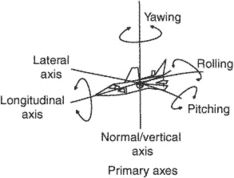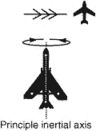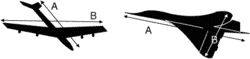# principal axis

Also found in: Dictionary, Thesaurus, Medical, Financial, Wikipedia.
Related to principal axis: centre of curvature

## principal axis

[′prin·sə·pəl ′ak·səs]
(crystallography)
The longest axis in a crystal.
(engineering acoustics)
A reference direction for angular coordinates used in describing the directional characteristics of a transducer; it is usually an axis of structural symmetry or the direction of maximum response.
(mathematics)
One of a set of perpendicular axes such that a quadratic function can be written as a sum of squares of coordinates referred to these axes.
For a conic, a straight line that passes through the midpoints of all the chords perpendicular to it.
For a quadric surface, the intersection of two principal planes.
(mechanics)
One of three perpendicular axes in a rigid body such that the products of inertia about any two of them vanish.
(optics)
McGraw-Hill Dictionary of Scientific & Technical Terms, 6E, Copyright © 2003 by The McGraw-Hill Companies, Inc.

## principal axisPrimary axesIn the illustration on the left, line A is the principal axis while in the illustration on the right, line B is the principal axis. The moment of inertia is maximum around these axes in these two cases.
i. The lateral, vertical, and longitudinal axes.
ii. The axis of relative wind.
iii. The principal inertial axis: that line passing through the center of gravity in a plane of symmetry about which a long, slender body tends to rotate when rolling.
iv. A rectilinear axis in the plane of a cross section of a structural member about which the moment of inertia is the maximum.
References in periodicals archive ?
The first one, namely [S.sub.1] = 0, corresponds to the manifold and indicates that the FFRB rotates about its principal axis III that coincides with the Z-axis.
Rotate the polygon by an angle-a bythe rotation to the vertices of the polygon in order to align the major principal axis with x-axis:
A point makes a high contribution to the inertia of a principal axis in two ways--when it has a large distance from the barycenter, even if it has a small mass, or when it has a large mass, but a small distance.
Initially, several exploratory factor analyses using principal axis factor analysis with oblimin rotation were conducted.
The factorial solutions found using principal axis, varimax rotation and principal components (with varimax or oblimin and [delta] = 0) also provided four factors.
The group consisted of African Americans, 3 European Americans and 1 Hispanic American, the principal Axis I diagnosis was conduct disorder (5), Oppositional Defiant Disorder (4) and Post Traumatic Stress Disorder (6).
Horizontal displacements were recorded for the internal index contour along the principal axis in the central part of structural scheme quarter; the scheme of measuring instruments location is given in Fig 4 b.
The factor extraction procedure was principal axis factoring.
The line joining the two centers is the principal axis of the lens.
For each principal axis the relevant eigenvector of orientation matrix was computed as the "most probable" orientation.
Up to 540 degrees rotation of the arm's principal axis has a unique "ball-track" soft-stop design that prevents arm damage common with conventional hard stops.

Site: Follow: Share:
Open / Close